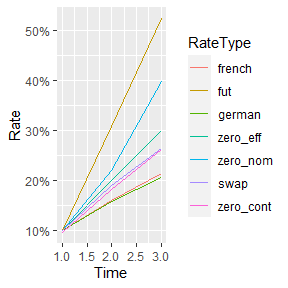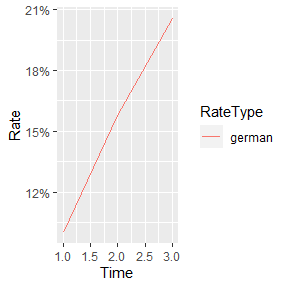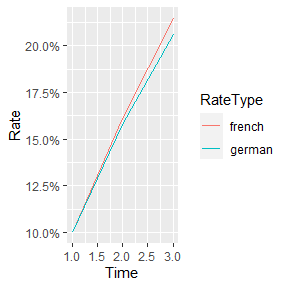# Intro to tvm

## Why tvm?

Well, first, tvm stands for “time value of money”, the usual name for financial calculations that involve interest rates, present values and future values.

Base R doesn’t have built-in calculations for this, and they are on of the most used functions in Excel for financial calculations. tvm attempts to provide these calculations to the user, using known Excel names where possible.

## Simple present values and rate calculations

For the Excel user, the following functions will be known

• npv
• xpnv
• irr
• xirr
• pmt
• rate

These work as expected, and require no further explanation. Please check the documentation or the README for some examples.

## Rate curves

A rate curve is an abstraction for a set of rates with different maturities. Currently, tvm only supports creating curves with equally spaced maturities, where the periodicity is implicitly specified by the user (i.e., no dates accepted).

In the current implementation, a rate curve is a S3 class, based on a list which has 2 components: a discount factor function $f, and a numeric vector$knots, which corresponds to the points of the curve where the bootstrapping between the different rate types is done.

The different rate types used are - zero (spot rates) in effective and nominal form (effective are compounded, nominal are linear) - fut (futures rate) - swap (bullet rates) - french (french type loans) - german (german type loans)

You create a rate curve with the constructor, and the use subsetting to get functions for the different loan structures. Note that only some rate types are available in the constructor.

The basis for all the curves are the discount factor. Within the constructor, calculations are performed to find the discount factors that create a curve equivalent to the one given.

You can create rate curves from a vector of rates (it is assumed that rates[i] is the rate corresponding to the i period), a rate function (given a maturity returns a rate) or a discount function (given a maturity returns a discount factor)

rate_curve(rates = c(0.1, 0.2, 0.3), rate_type = "zero_eff")
## $f ## function (x, deriv = 0, extrapol = c("linear", "cubic")) ## { ## extrapol <- match.arg(extrapol) ## deriv <- as.integer(deriv) ## if (deriv < 0 || deriv > 3) ## stop("'deriv' must be between 0 and 3") ## i <- findInterval(x, x0, all.inside = (extrapol == "cubic")) ## if (deriv == 0) ## interp <- function(x, i) { ## h <- dx[i] ## t <- (x - x0[i])/h ## t1 <- t - 1 ## h01 <- t * t * (3 - 2 * t) ## h00 <- 1 - h01 ## tt1 <- t * t1 ## h10 <- tt1 * t1 ## h11 <- tt1 * t ## y0[i] * h00 + h * m[i] * h10 + y0[i + 1] * h01 + ## h * m[i + 1] * h11 ## } ## else if (deriv == 1) ## interp <- function(x, i) { ## h <- dx[i] ## t <- (x - x0[i])/h ## t1 <- t - 1 ## h01 <- -6 * t * t1 ## h10 <- (3 * t - 1) * t1 ## h11 <- (3 * t - 2) * t ## (y0[i + 1] - y0[i])/h * h01 + m[i] * h10 + m[i + ## 1] * h11 ## } ## else if (deriv == 2) ## interp <- function(x, i) { ## h <- dx[i] ## t <- (x - x0[i])/h ## h01 <- 6 * (1 - 2 * t) ## h10 <- 2 * (3 * t - 2) ## h11 <- 2 * (3 * t - 1) ## ((y0[i + 1] - y0[i])/h * h01 + m[i] * h10 + m[i + ## 1] * h11)/h ## } ## else interp <- function(x, i) { ## h <- dx[i] ## h01 <- -12 ## h10 <- 6 ## h11 <- 6 ## ((y0[i + 1] - y0[i])/h * h01 + m[i] * h10 + m[i + 1] * ## h11)/h ## } ## if (extrapol == "linear" && any(iXtra <- (iL <- (i == 0)) | ## (iR <- (i == (n <- length(x0)))))) { ## r <- x ## if (any(iL)) ## r[iL] <- if (deriv == 0) ## y0[1L] + m[1L] * (x[iL] - x0[1L]) ## else if (deriv == 1) ## m[1L] ## else 0 ## if (any(iR)) ## r[iR] <- if (deriv == 0) ## y0[n] + m[n] * (x[iR] - x0[n]) ## else if (deriv == 1) ## m[n] ## else 0 ## ini <- !iXtra ## r[ini] <- interp(x[ini], i[ini]) ## r ## } ## else { ## interp(x, i) ## } ## } ## <bytecode: 0x00000209a1afa188> ## <environment: 0x00000209a1727040> ## ##$knots
##  1 2 3
##
## $functor ## function (x, y) ## splinefun(x = x, y = y, method = "monoH.FC") ## <environment: 0x00000209a1df0890> ## ##$rate_scale
##  1
##
## attr(,"class")
##  "rate_curve"
rate_curve(fun_r = function(x) rep_len(0.1, length(x)), rate_type = "swap", knots = 1:10)
## $f ## function (x, deriv = 0, extrapol = c("linear", "cubic")) ## { ## extrapol <- match.arg(extrapol) ## deriv <- as.integer(deriv) ## if (deriv < 0 || deriv > 3) ## stop("'deriv' must be between 0 and 3") ## i <- findInterval(x, x0, all.inside = (extrapol == "cubic")) ## if (deriv == 0) ## interp <- function(x, i) { ## h <- dx[i] ## t <- (x - x0[i])/h ## t1 <- t - 1 ## h01 <- t * t * (3 - 2 * t) ## h00 <- 1 - h01 ## tt1 <- t * t1 ## h10 <- tt1 * t1 ## h11 <- tt1 * t ## y0[i] * h00 + h * m[i] * h10 + y0[i + 1] * h01 + ## h * m[i + 1] * h11 ## } ## else if (deriv == 1) ## interp <- function(x, i) { ## h <- dx[i] ## t <- (x - x0[i])/h ## t1 <- t - 1 ## h01 <- -6 * t * t1 ## h10 <- (3 * t - 1) * t1 ## h11 <- (3 * t - 2) * t ## (y0[i + 1] - y0[i])/h * h01 + m[i] * h10 + m[i + ## 1] * h11 ## } ## else if (deriv == 2) ## interp <- function(x, i) { ## h <- dx[i] ## t <- (x - x0[i])/h ## h01 <- 6 * (1 - 2 * t) ## h10 <- 2 * (3 * t - 2) ## h11 <- 2 * (3 * t - 1) ## ((y0[i + 1] - y0[i])/h * h01 + m[i] * h10 + m[i + ## 1] * h11)/h ## } ## else interp <- function(x, i) { ## h <- dx[i] ## h01 <- -12 ## h10 <- 6 ## h11 <- 6 ## ((y0[i + 1] - y0[i])/h * h01 + m[i] * h10 + m[i + 1] * ## h11)/h ## } ## if (extrapol == "linear" && any(iXtra <- (iL <- (i == 0)) | ## (iR <- (i == (n <- length(x0)))))) { ## r <- x ## if (any(iL)) ## r[iL] <- if (deriv == 0) ## y0[1L] + m[1L] * (x[iL] - x0[1L]) ## else if (deriv == 1) ## m[1L] ## else 0 ## if (any(iR)) ## r[iR] <- if (deriv == 0) ## y0[n] + m[n] * (x[iR] - x0[n]) ## else if (deriv == 1) ## m[n] ## else 0 ## ini <- !iXtra ## r[ini] <- interp(x[ini], i[ini]) ## r ## } ## else { ## interp(x, i) ## } ## } ## <bytecode: 0x00000209a1afa188> ## <environment: 0x00000209a4ddb2c8> ## ##$knots
##    1  2  3  4  5  6  7  8  9 10
##
## $functor ## function (x, y) ## splinefun(x = x, y = y, method = "monoH.FC") ## <environment: 0x00000209a4dcaad8> ## ##$rate_scale
##  1
##
## attr(,"class")
##  "rate_curve"
rate_curve(fun_d = function(x) 1 / (1 + x), knots = 1:10)
## $f ## function(x) 1 / (1 + x) ## ##$knots
##    1  2  3  4  5  6  7  8  9 10
##
## $functor ## function (x, y) ## splinefun(x = x, y = y, method = "monoH.FC") ## <environment: 0x00000209a4e462b0> ## ##$rate_scale
##  1
##
## attr(,"class")
##  "rate_curve"

The subset operator allows you to retrieve certain rates only, or retrieve the equivalent rate curve in another type

r <- rate_curve(rates = c(0.1, 0.2, 0.3), rate_type = "zero_eff")
r[, c(1, 2)]
##  0.1 0.2
r["zero_eff"]
## function (x)
## rescale(f(x), rate_scale = r\$rate_scale, rate_type = rate_type)
## <bytecode: 0x00000209a5372878>
## <environment: 0x00000209a53e21b8>
r["swap",c(1.5, 2)]
##  0.1463039 0.1905512

Plotting rate curves is supported, and you can choose which rate type or types to plot

plot(r)plot(r, rate_type = "german")plot(r, rate_type = c("french", "german"))